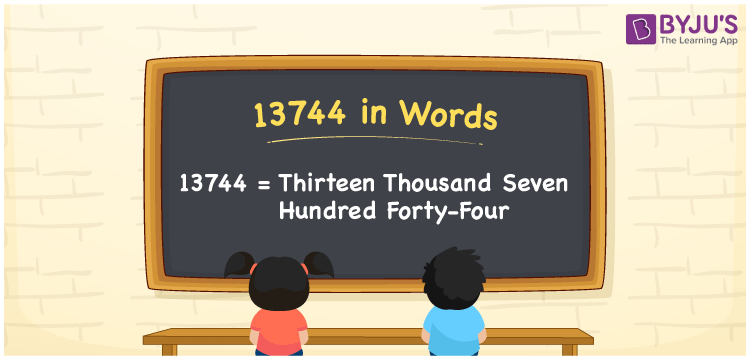# 13744 in Words

13744 in words is written as Thirteen thousand seven hundred forty-four. In both the International System of Numerals and the Indian System of Numerals, 13744 is written as Thirteen thousand seven hundred forty-four. The number 13744 is a Cardinal Number as it denotes some quantity. For example, “that mobile phone costs 13744 rupees”.

 13744 in Words Thirteen thousand seven hundred forty-four Thirteen thousand seven hundred forty-four in Number 13744

## 13744 in English Words

13744 in English words is read as “Thirteen thousand seven hundred forty-four”.## How to Write 13744 in Words?

To write 13744 in words, we shall use the place value chart. In the place value chart, put 1 in the ten thousands, 3 in the thousands, 7 in the hundreds, 4 in the tens and ones, respectively. Let us make a place value chart to write the number 13744 in words.

 Ten Thousands Thousands Hundreds Tens Ones 1 3 7 4 4

Thus, we can write the expanded form as

1 × Ten Thousand + 3 × Thousand + 7 × Hundred + 4 × Ten + 4 × One

= 1 × 10000 + 3 × 1000 + 7 × 100 + 4 × 10 + 4 × 1

= 10000 + 3000 + 700 + 40 + 4

= 13744

= Thirteen thousand seven hundred forty-four.

13744 is a natural number, the successor of 13743 and the predecessor of 13745.

13744 in words – Thirteen thousand seven hundred forty-four

• Is 13744 an odd number? – No
• Is 13744 an even number? – Yes
• Is 13744 a perfect square number? – No
• Is 13744 a perfect cube number? – No
• Is 13744 a prime number? – No
• Is 13744 a composite number? – Yes

## Frequently Asked Questions on 13744 in Words

Q1

### How to write 13744 in words?

13744 in words is written as Thirteen thousand seven hundred forty-four.
Q2

### How to write 13744 in the International and Indian System of Numerals?

In both, the system of numerals, 13744 in words, is written as Thirteen thousand seven hundred forty-four.
Q3

### How to write 13744 in a place value chart?

In the place value chart, write 1 in the ten thousands, 3 in the thousands, 7 in the hundreds, 4 in the tens and ones, respectively.